Reactive Power Management using Firefly and Spiral Optimization under Static and Dynamic Loading Conditions | OMICS International
Journal of Electrical & Electronic Systems

# Reactive Power Management using Firefly and Spiral Optimization under Static and Dynamic Loading Conditions

Ripunjoy Phukan*

Indian Institute of Technology, Guwahati, India

*Corresponding Author:
Ripunjoy Phukan
Indian Institute of Technology, Guwahati, India
Tel: 91-80118-10978
E-mail: [email protected]

Received Date: September 08, 2013; Accepted Date: November 28, 2013; Published Date: December 02, 2013

Citation: Phukan R (2013) Reactive Power Management using Firefly and Spiral Optimization under Static and Dynamic Loading Conditions. J Electr Electron Syst 2: 114. doi:10.4172/2332-0796.1000114

Copyright: © 2013 Phukan R, et al. This is an open-access article distributed under the terms of the Creative Commons Attribution License, which permits unrestricted use, distribution, and reproduction in any medium, provided the original author and source are credited.

Visit for more related articles at Journal of Electrical & Electronic Systems

#### Abstract

Power System planning encompasses the concept of minimization of transmission losses keeping in mind the voltage stability and system reliability. Voltage profile decides the state of a system and its control is dependent on Generator source voltage, shunt/series injection, transformer taps etc. Optimal parameter setting in system level is needed for managing the available resources economically. This paper presents the use of Firefly and Spiral optimization as novel schemes for minimizing the active power loss along with partial compensation of inter bus voltage drop. The objective function has been evaluated under both static and dynamic loading conditions. The control variables being generator bus voltage, capacitor shunts and transformer taps. These methods were employed in an IEEE 6-bus system and the results were tabulated.

#### Introduction

Reactive power optimization problem is a non-linear combinatorial optimization problem. This problem came to focus on account of the majority of loads being reactive in nature. The modern power system is usually controlled by a monopoly resulting in freedom of action and better controllability of generator voltage levels, shunt capacitors and transformer taps (vertical integration). Under stable running conditions the devices are operational at rated values and have minimal stress. However, a static condition is expected to stay for a maximum duration of 10 minutes. During dynamic loading, the optimal parameters must be incorporated into the system to curtail minimum losses and prevent a power outage.

Shunt injection using reactive sources, such as a capacitor, creates phase advancement in line voltage. Assuming lossless lines, the resistance is assumed zero, creating a purely inductive line. Transformer taps also cause the necessary stepping of voltages near stressed lines (Lines where the thermal limits are easily attained on account of loading). Research into accurate location of such transformers has been shown in the study of Kargarian and Raoofat . The positioning in this paper is assumed fixed, i.e. the system is functional since a long time period, and relocation may add to costs. One advantage for using compensators is the absence of fuel costs, while generator scheduling adds to fuel costs as well. However in this paper the economic aspect of VAR Compensation is neglected. The efficiency of Shunt VAR Compensators, Thyristor Switched Capacitors, Thyristor Controlled Reactors have proved the validity in the use of FC-TCR elements in reactive voltage control. STATCOM devices utilize switching strategies for controlling reactive power flow through a bridged circuit, as proposed in the study of Singh and Elmoursi [2,3].

The conventional methods of LaGrange’s Multipliers prove incompatible with these applications due to stagnation and localization of optimum solutions . Some of these techniques include linear programming, non-linear programming, mixed integer method, decomposition method. In recent years some Artificial Intelligence methods such as expert systems, neural networks and simulated annealing have been developed. The modern optimization techniques fall under the genre of Artificial Intelligence, where the natural mimicry is postulated in the form of equations. Several such techniques have been stated in . Previous works on Reactive Power Planning include the application of Genetic Algorithms , followed by Particle Swarm Optimization [7,8]. Genetic-Simulated annealing and interior point method  along with adaptive PSO  based on optimal control principle were a few improvisations. Other approaches incorporate the usage of load forecasting using Radial Basis functions  and successive quadratic programming methods . These methods have proven to be effective and are useful in their own versions. Al-alawi, et al.  have presented an ANN based technique for tuning SVCs in a power system. Significant amount of literature survey indicates that ANN and deregulated conditions have been enforced in [14-16]. Hybridized market and congestion management have been invoked in [17-19]. Voltage control area reserves and wind power volatility have been dealt with in papers  and .

The Firefly Optimization invokes the luminescent behavior of fireflies . Earlier works with fireflies include the Economic Load Dispatch problem . Spiral optimization is a latest technique introduced by Kenichi Tamura . It interprets the spiral phenomenon in nature such as hurricanes and whirling currents. These techniques have been applied to a static IEEE-6 bus system with partial compensation. The system architecture is similar to . The concept of compensation has been additionally introduced in the fitness function. Further calculations were done for a dynamic system as well [25,26]. A comparison between both the algorithms has been given and the success of spiral optimization as a potent Reactive Power Optimization tool has been justified. The following two sections (4 and 5) deal with the algorithms employed in detail. This is followed by the VAR optimization method in section 6 with results and tabulation in 7. Finally, the Conclusion is derived in Section 8.

#### Firefly Optimization

Fireflies

This optimization algorithm can be used for solving real valued multi-modal optimization problems. It was first proposed by D. Ghose in 2006. If there are no fireflies brighter than a given firefly, it will move randomly. Fireflies use the concept of path identification based on light intensity from another firefly. Being a stochastic algorithm, it exploits all possible regions of the search space for information. This algorithm was inspired by the motion of fireflies and glow-worms in search for a mate, based on their luminescence behavior. Naturally, a firefly would move in the direction of maximum brightness indicating the most feasible mate. The light intensity (brightness) is given by equation (1). It varies exponentially with the distance between two fireflies and is irrespective of their sex. As the attractiveness of fireflies is proportional to the light intensity, similar variations are found between attractiveness and distance (2). In practical problems the light intensity is determined by the landscape of the objective function.

I = I0.e-γr (1)

β = β0.e-γr (2)

The movement of fireflies towards one another is governed by equation (3). For most cases in our implementation we take γ as 1.0 and β0 as 1.0.

xi = xi+ 2 β .e-γr .(xj-xi)+α.Єi (3)

where, xi is the current position in 1 Dimension and xi is the previous best position of the fireflies. The following update is carried over all the dimensions. The parameter α.Єi is a user defined bias control to make corrections in deviations from the optimal value on account of external disturbances. The algorithm for Fireflies is expressed in Figure 1.

#### Spiral Optimization

Spiral phenomenon

The focused spiral phenomenon is approximated to logarithmic spirals which frequently appear in nature. For example, the Nautilus shell, hurricanes and whirlpools. These spirals are associated with gradually decreasing radius as the vector rotates with reference to the previous points. A 2-dimensional model uses the rotation vector as given in equation (4). The movement of particles is described by equation (6). For a greater degree of search space exploitation, the angle, θ, can be increased while for concentration over a smaller region it can be decreased. Similar variations can be done with the radius as well.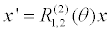(4)

where, rotation matrix is given by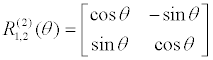For an ‘n’ dimensional system the rotation matrix is modified as shown by (5),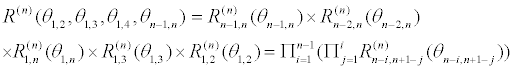(5)

where, 0 < θi,j < 2π are rotation angles each plane around the origin at every k, 0 < r < 1 is the convergence rate between any point and the optimal solution. The positional update is given by equation (6).

x(k+1)=Sn(r,θ)x(k)–( Sn(r,θ)–In)x* (6)

where, In is the identity matrix of order (n x n) and x* is the previous best global solution.

Algorithm in N dimensions

Step 0: [Preparation]: Select the number of search points m ≥ 2, the parameters 0 < θ < 2π, 0 < r < 1, of Sn(r,θ), and the maximum number of iterations kmax with k = 0.

Step 1: [Initialization]: Set the initial points xi(0) Є Rn, i=1,2,3,… ….,m in the feasibility region at random and the center x* as x* = xig(0), ig = arg minif(xi(0)), i = 1,2,…,m.

Step 2: [Updating xi]: X is updated as per equation (6).

Step 3: [Updating x*]: x* = xig(k+1), ig = arg minif(xi(k+1)), i = 1,2,…,m.

Step 4: [Checking termination criteria]: If k = kmax then terminate. Otherwise set k = k + 1, and return to Step 2.

The spiral phenomenon from equation (4) for r = 0.95 and θ = π/4 is as shown in Figure 2. As observed both these algorithms have the potential for attaining a minimum, with parameter optimization. This phenomenon is also termed as combinatorial optimization. Further, it will be shown that these algorithms have the capability to converge within lesser iteration count. So, for dynamic processes these algorithms prove efficient.

#### Problem Formulation

Reactive power scheduling is usually carried out every ten minutes to one hour . It consists of the optimization at each discrete time instant k of a power system characterized by operational conditions r(k), which represent the scheduled load demand, active power generation pattern, and network topology at instant k.The main objective of the Reactive Power Optimization problem is to minimize the active power loss by means of controlling the Generator real power outputs, Shunt capacitor banks and the transformer tap positions. An-online simulation during dynamic conditions can prove to be ineffective due to time constraint. So, offline studies are done to mitigate security problems in future avoiding grid collapse or blackouts. A repeated simulation for various loading conditions can be done to create an adaptive look up table that eases the process of decision making. For the sake of convenience we have assumed the generator and load buses to be loaded to 1.2 times of their base values. These parameters are the positional co-ordinates in our optimization algorithm and are updated after every iteration. The bus voltages are calculated by means of a suitable load flow analysis. The scope of the problem is enhanced when we introduce several operating constraints in the problem. The transformer taps, voltage magnitudes and the reactive shunt compensation must be within the prescribed limits. The equality constraints are automatically satisfied using load flow analysis, while the inequality constraints need to be satisfied using randomization in optimization algorithms. Another possible solution would be to increase the step size in parameter movement.

The static loading condition occurs in a system when it is operating under normal state. The active real power losses in this system are given by equation (7).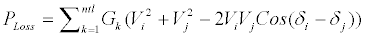(8)

The constraints involved are shown below: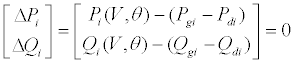where,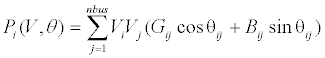and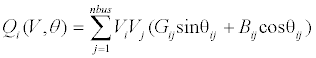i Є n, where set of buses except the swing bus.

(2) Bus voltage magnitude constraints:

Vi,min≤ Vi ≤ V i,max (9)

i Є number of buses

(3) Generator bus reactive power constraints:

QGi-min < QGi < QGi-max (10)

i Є number of PV buses

(4) Reactive power source capacity constraints:

Qsh-min < Qsh-i < Qsh-max (11)

i Є number of buses with shunt capacitors

(5) Transformer taps position constraints:

Ti-min ≤ Ti ≤ Ti-max (12)

i Є number of transmission lines with OLTC transformers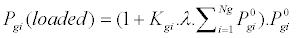(13)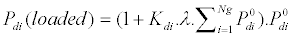(14)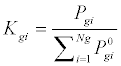(15)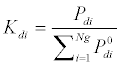(16)

Compensation using OLTC transformers

In general a tap changing transformer is placed between any two buses for reducing the voltage drop and hence, the losses. This method has gained massive potential due to its equivalence with an auto-transformer. This arrangement works best if the voltages at the two ends are equal. However, a practical condition will always show different voltages at the two ends. So the system is said to be partially compensated. With this in mind, equation (17) holds true. V1 and V2 are obtained from the optimization algorithm and the transformer tap positions are checked directly from equation (17). The resulting losses are given by equation (18) (Figure 3).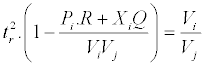(17)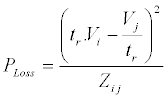(18)

V1 and V2 are the nominal voltages at the ends of the line and the actual voltages being ts V1 and trV1. The tap changing ratios are used to partially compensate the system. As tstr is made equal to unity it ensures that the overall voltage level remains in the same order. The impedance of the line is R+jX.

Parameters:

Ploss = System loss (Fitness Function)

Vi = Voltage at bus ‘i’

Vj = Voltage at bus ‘j’

δi = Phase angle of Vi

δi = Phase angle of Vj

PGi = Bus ‘i’ real power supply

QGi = Bus ‘i’ reactive power supply

PDi = Bus ‘i’ real power load

QDi = Bus ‘i’ reactive power load

Gij = Mutual conductance between bus i and j

Bij = Mutual susceptance between bus i and j

θij = Phase angle difference between buses ‘i’ and ‘j’

Vi,min ,Vi,max = Bus ‘i’ voltage limits

QGi-min ,QGi-max = Bus ‘i’ reactive power limits

Qsh-min, Qsh-max = Shunt reactive power limits

Ti-min, Ti-max = Transformer ‘i’ tap position limits

Ti = transformer tap position

Qsh,i = Bus ‘i’ shunt reactive power

With compensation and dynamic loading the overall fitness function is given by: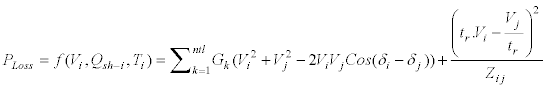(18)

In the above equation, the real power losses between nodes ‘i’ and ‘j’ must be neglected and reproduced in the form of the second term in the expression.

#### Results and Discussion

An IEEE 6 bus system is considered and the optimal value for generation and transformer taps is tabulated. The minimum loss is obtained from the fitness function (19). The IEEE 6 bus system is shown in Figure 4. The standard IEEE 6 bus system data was taken from the work of Tamura and Yasuda  (Tables 1 and 2). The figure was made in Power System Simulator for clarity.

Figure 4: IEEE 6 bus system with bus 1 as the slack bus, bus 2 as the generator bus and the remaining buses are the load buses. Rated values are mentioned in the figure.

LSB LTB R (pu) X (pu) OLTC
1 6 0.123 0.518 1.0
1 4 0.08 0.370 1.0
4 6 0.097 0.407 1.0
6 5 0.000 0.300 1.025
5 2 0.282 0.320 1.0
2 3 0.723 1.050 1.0
4 3 0.000 0.133 1.1

Table 1: System Line Data.

Bus No. Vm (pu) Pd
(pu)
Qd
(pu)
Qg min (pu) Qg max
(pu)
Qsh
(pu)
1 1.05 0 0 0 0 0
2 1.1 0 0 -0.4 0.5 0
3 1.0 0.275 0.065 0 0 0
4 1.0 0 0 0 0 0
5 1.0 0.15 0.09 0 0 0
6 1.0 0.25 0.005 0 0 0

Table 2: System Bus Data.

The optimization parameter results for a static and a dynamic system have been tabulated in Tables 3 and 4 respectively. The optimization parameter results for a static and a dynamic compensated system have been tabulated in Tables 5 and 6 respectively.

Variable Lower limit Upper limit Firefly
(pu)
Spiral
(pu)
VG1 1.000 1.100 1.0054 1.0157
VG2 1.100 1.150 1.1173 1.1224
QSH 1 0.000 0.050 0.035 0.0183
QSH 2 0.000 0.055 0.0044 0.0069

Table 3: Optimization Results.

Variable Lower limit Upper limit Firefly
(pu)
Spiral
(pu)
VG1 1.000 1.100 1.0064 1.0005
VG2 1.100 1.150 1.1023 1.1088
QSH 1 0.000 0.050 0.0009 0.0248
QSH 2 0.000 0.055 0.0131 0.0345

Table 4: Optimization Results.

Variable Lower limit Upper limit Firefly
(pu)
Spiral
(pu)
VG1 1.000 1.100 1.0979 1.0921
VG2 1.100 1.150 1.1472 1.1130
QSH 1 0.000 0.050 0.0361 0.0475
QSH 2 0.000 0.055 0.0033 0.0013
T43 0.9 1.1 1.0216 1.0229
T56 0.9 1.1 1.0353 1.0342

Table 5: Optimization Results.

Variable Lower limit Upper limit Firefly
(pu)
Spiral
(pu)
VG1 1.000 1.100 1.0026 1.0903
VG2 1.100 1.150 1.1244 1.1487
QSH 1 0.000 0.050 0.0440 0.0548
QSH 2 0.000 0.055 0.0069 0.0042
T43 0.9 1.1 1.0229 1.0239
T56 0.9 1.1 1.0359 1.0351

Table 6: Optimization Results.

The load flow study was conducted using Gauss Siedal method for 5 iterations. β0 was taken as any random variable between (0 and 1). Spiral and F.A. have been run for 100 iterations each. λ has been taken as 0.2. The net power loss is tabulated for all the cases considered in Table 7.

Test Case Firefly
(MW)
Spiral
(MW)
1. Static system without compensation 4.56 4.53
2. Static system with compensation 4.52 4.43
3. Dynamic system without compensation 5.56 5.49
4. Dynamic system with compensation 5.4 5.36

Table 7: Optimum System Loss.

The convergence characteristic of these algorithms is studied and their fitness is plotted with respect to the iteration count. Firefly algorithm is simulated by including a random walk module in the original algorithm (Figure 5). The Spiral algorithm is run for 100 iterations under uncompensated static conditions Figure 6 and a comparison between F.A and Spiral has been made in Figure 7. The N.R. load flow is done for the base case  as well as the optimal parameters for static compensated system. The results are shown in Tables 7 and 8. The convergence criterion is taken as 0.000001. Acceptable tolerance is to be greater than 0.000001. All the results have been simulated using MATLAB Software (version 7.0). Separate coding has been done for N.R, Gauss Siedal, Spiral and Firefly algorithms with all the test cases considered.

Normal
(MW)
Firefly
(MW)
Spiral
(MW)
5.358 5.264 4.89

Table 8: Optimum System Loss.

From Bus To Bus P (MW) Q
(MVAR)
Real Power Loss Reactive Power loss
1 6 10.99 2.21 0.13 0.546
1 4 16.39 -34.5 0.979 4.527
2 3 14.6 -10 0.183 2.655
2 5 30.4 -15.39 2.643 0.6
3 4 -13.08 6.43 0 0.19
4 6 2.33 37.63 0.958 4.021
6 5 -12.75 9.07 0 0.62

Table 9: Line Flow Data for Spiral Algorithm.

The discrepancy between the values of Tables 7 and 9 is indicative of the fact that the Gauss Siedal method computes the active losses with greater precision for a 6 bus system. However, as the size of the network increases, N.R. method is more favorable.

Both the techniques adopted have successfully allocated the parameters within the optimal conditions (Tables 3-6). As observed from Table 7, Spiral algorithm provides better optimal values than F.A. under all the test cases considered. As shown in Figure 7, Spiral curve is going under the firefly graph during the later course of the iterations. As expected, Compensation reduces the losses by an appreciable 0.1 MW in most of the cases Table 7. Eventually for huge power systems this value can be in the order of tens or hundreds. One special feature observed is that both the algorithms attain fast convergence between 9 and 10 iterations. This means that for discrete time step of 0.005 seconds the scheduling will occur in 0.05 seconds itself (considering 10 iterations). Moreover, F.A. could be enhanced by using random walk simulation (Figure 5) to increase the possibility of a better solution. But, that feature is not used as it violates the inequality constraints and increases the computation time. A further study is done using Newton Raphson method, for a static compensated system (Table 9). Spiral Optimization proved better in that regard as well giving a minimal real power loss of 4.89 MW. From the line flow data it is shown that real power loss between buses 3-4 and 5-6 have been compensated due to the presence of transformer taps at those locations (Figure 4).

Figure 7: Comparison between spiral and firefly optimization for 10 iterations under static uncompensated system.

#### Conclusion

Reactive power should be properly controlled and applied to maintain system balance. It is the responsibility of the utilities to effectively control and plan the reactive power losses along with the financial expenses. Since the concept here involves the operation of a centralized TSO, effective communication channels for processing data is mandatory. Spiral optimization is a promising meta heuristic technique based on random sampling of search space. It is comparable to other evolutionary algorithms such as Firefly algorithm with even better performance. The ability of spiral to converge to the desired location with logarithmic convergence is suitable for Reactive Power Planning. Furthermore, Gauss Siedal analysis proved to be a better option for load flow compared to N.R. method for a 6 bus system. Partial compensation with tap setting transformers can be successfully implemented in the power system to avoid voltage drop between buses where critical loads have been stationed. This will reduce thermal stress and avoid systemic outages. The ability of both these algorithms to react within short epoch duration justifies their significance in a dynamic simulation platform.

#### References

Select your language of interest to view the total content in your interested language

### Article Usage

• Total views: 12648
• [From(publication date):
December-2013 - Dec 16, 2019]
• Breakdown by view type
• HTML page views : 8810Can't read the image? click here to refresh